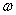Courses

# Test: Progressive Waves

## 10 Questions MCQ Test Physics For JEE | Test: Progressive Waves

Description
This mock test of Test: Progressive Waves for JEE helps you for every JEE entrance exam. This contains 10 Multiple Choice Questions for JEE Test: Progressive Waves (mcq) to study with solutions a complete question bank. The solved questions answers in this Test: Progressive Waves quiz give you a good mix of easy questions and tough questions. JEE students definitely take this Test: Progressive Waves exercise for a better result in the exam. You can find other Test: Progressive Waves extra questions, long questions & short questions for JEE on EduRev as well by searching above.
QUESTION: 1

### Waves associated with moving protons, electrons, neutrons, atoms are known as

Solution:

Matter waves are a central part of the theory of quantum mechanics, being an example of wave–particle duality. All matter exhibits wave-like behavior. For example, a beam of electrons can be diffracted just like a beam of light or a water wave.
Matter is made of atoms, and atoms are made protons, neutrons and electrons. These are not macroscopic particles.

QUESTION: 2

### Maximum destructive inference between two waves occurs when the waves are out of the phase by

Solution:

Let the waves be
y1 = Asin(wt)
y2 = Asin(wt + φ)
If φ = π the interference is destructive.
Destructive interference occurs when the maxima of two waves are 180 degrees out of phase: a positive displacement of one wave is cancelled exactly by a negative displacement of the other wave. The amplitude of the resulting wave is zero. The dark regions occur whenever the waves destructively interfere.
Hence B is the correct answer.

QUESTION: 3

### In what types of waves can we find capillary waves and gravity waves?

Solution:

A longer wavelength on a fluid interface will result in gravity–capillary waves which are influenced by both the effects of surface tension and gravity, as well as by fluid inertia. On the open ocean, much larger ocean surface waves (seas and swells) may result from coalescence of smaller wind-caused ripple-waves.

QUESTION: 4

To the nearest order of magnitude, how many times greater than the speed of sound is the speed of light?

Solution:

Speed of sound in air is 343 m/s or we can say approx 300m/s
And speed of light is approx 300,000,000 m/s
Clearly the ratio is 106

QUESTION: 5

The angular frequencyis related to the time period T by

Solution:

Angular frequency is associated with the number of revolutions an object performs in a certain unit of time. In that sense is related to frequency but in terms of how many times it turns a full period of motion in radians units.
The formula of angular frequency is given by:
Angular frequency = 2 π / (period of oscillation)
ω = 2π / T = 2πf
Where we have:
ω: angular frequency
T: period
f: frequency
Hence C is correct.

QUESTION: 6

A steel wire 120 cm long has mass 12 g attached to it. If the wire is under a tension of 150N, what is the speed of transverse wave in the wire?

Solution:
QUESTION: 7

In a wave which quantity is transferred from one point to the other.

Solution:

'Wave' is a common term for a number of different ways in which energy is transferred: In electromagnetic waves, energy is transferred through vibrations of electric and magnetic fields. In sound waves, energy is transferred through vibration of air particles or particles of a solid through which the sound travels.

QUESTION: 8

What is the range of frequency of audible sound?

Solution:

20 to 20,000 Hz
The SI unit of audio frequency is the hertz (Hz). It is the property of sound that most determines pitch. The generally accepted standard range of audible frequencies for humans is 20 to 20,000 Hz, although the range of frequencies individuals hear is greatly influenced by environmental factors.

QUESTION: 9

In a transverse wave, the constituents of the medium oscillate ___________to the direction of wave propagation and in a longitudinal wave they oscillate ________to the direction of propagation.

Solution:

Both transverse and longitudinal waves are mechanical waves where they need material medium to propagate. The motion of transverse wave is perpendicular to the direction of the wave motion, and parallel for longitudinal waves.

QUESTION: 10

The ratio of velocity of sound in hydrogen and oxygen at STP is __________

Solution:

vH/vO = √(MO/MH)
=√(32/2)=4:1.